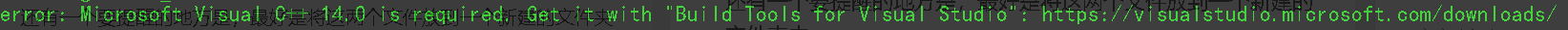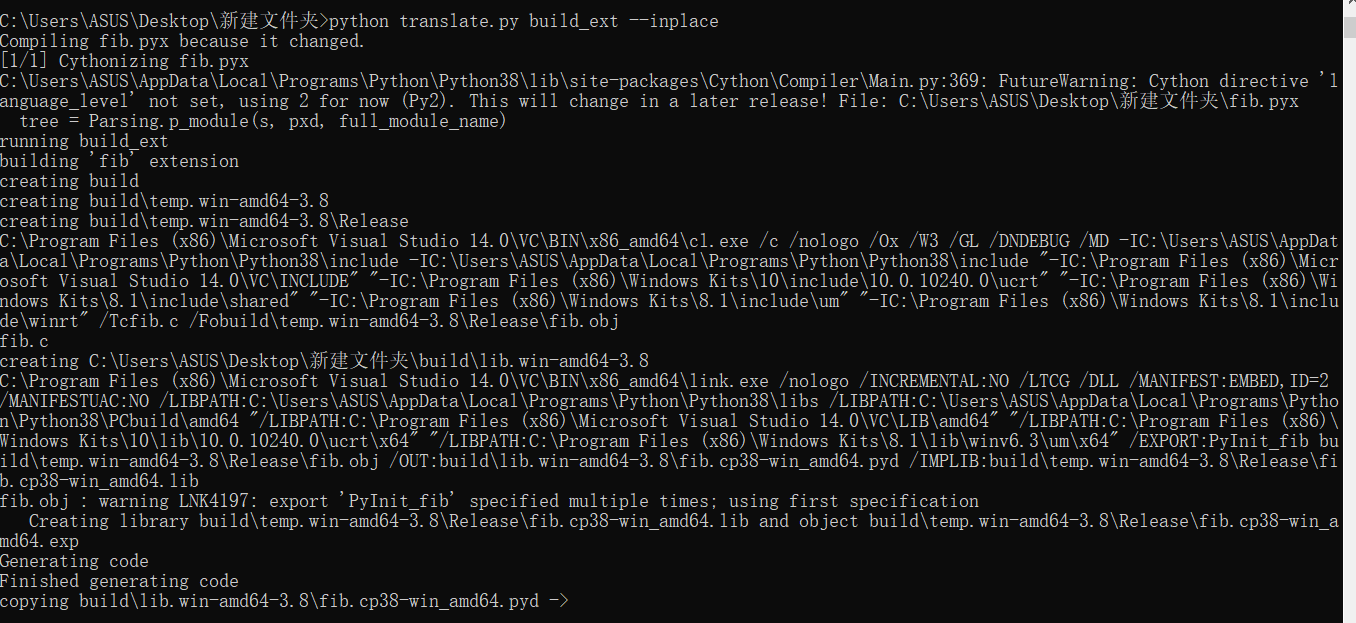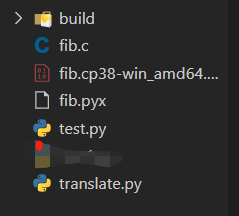# 将python代码转化为c语言代码，提高运行效率

``````pip install cython
``````
• 1

``````def fib(n):
return 1 if n ==1 or n == 2 else fib(n - 1) + fib(n - 2)
``````
• 1
• 2

``````from setuptools import setup
from Cython.Build import cythonize

setup(ext_modules=cythonize('fib.pyx'))
``````
• 1
• 2
• 3
• 4

``````python translate.py build_ext --inplace
``````
• 1``````import time
from fib import fib

start = time.time()
result = fib(20)
end = time.time()
print('斐波那契数列第20项为'+ str(result)+'耗时：'+str(end - start)+'秒')
``````
• 1
• 2
• 3
• 4
• 5
• 6
• 7``````斐波那契数列第40项为102334155耗时：5.765635251998901秒
``````
• 1

``````import time

def fib(n):
return 1 if n ==1 or n == 2 else fib(n - 1) + fib(n - 2)

start = time.time()
result = fib(40)
end = time.time()
print('斐波那契数列第20项为'+ str(result)+'耗时：'+str(end - start)+'秒')
``````
• 1
• 2
• 3
• 4
• 5
• 6
• 7
• 8
• 9
``````斐波那契数列第40项为102334155耗时：27.247100830078125秒
``````
• 1